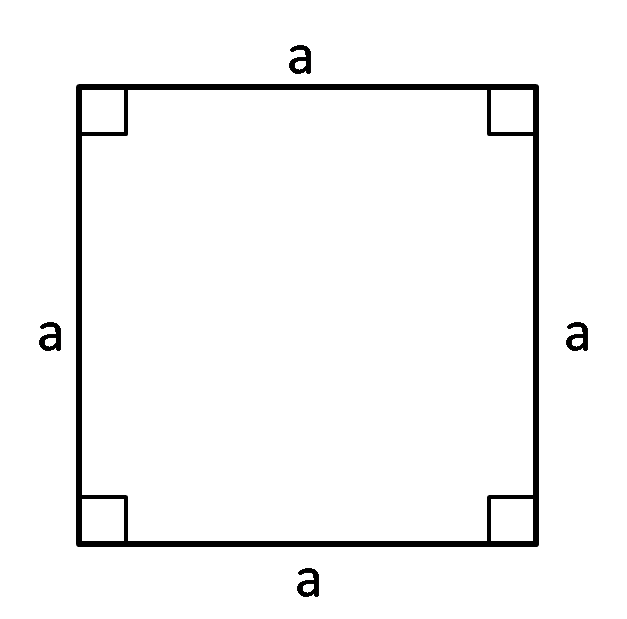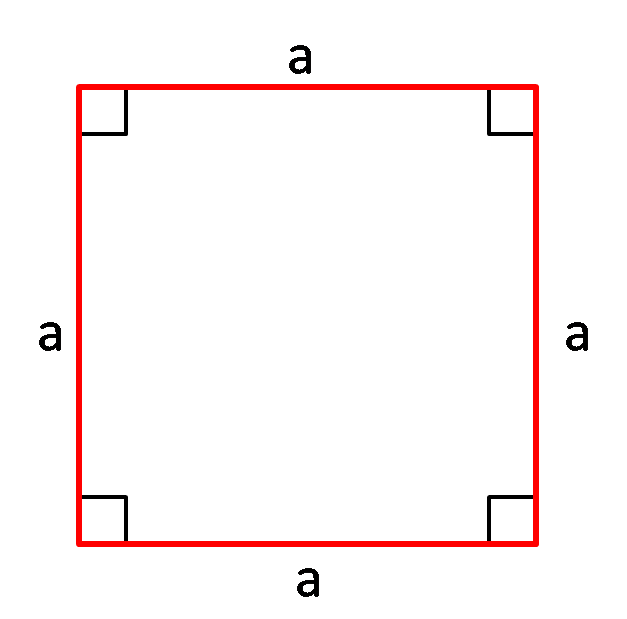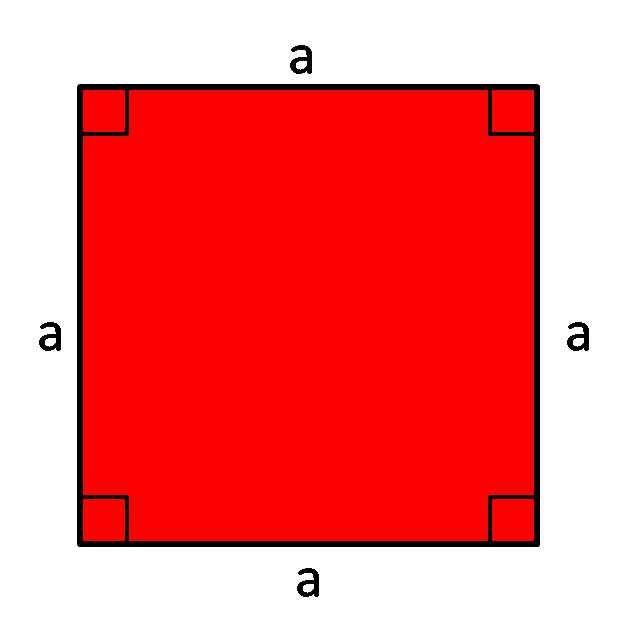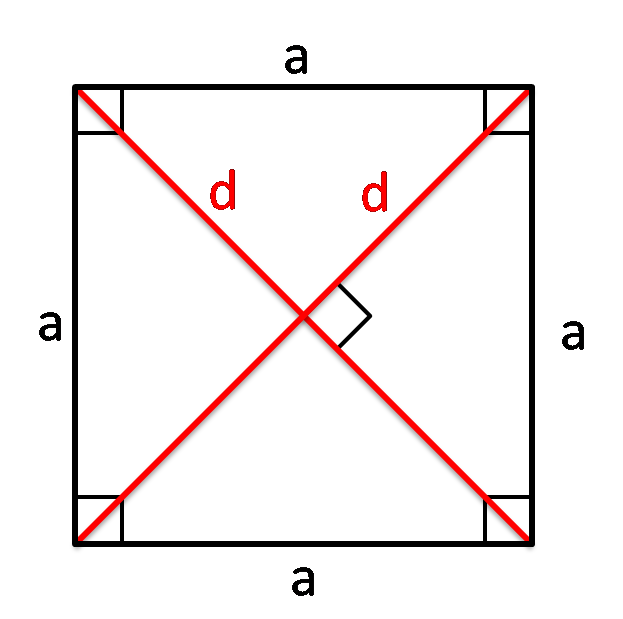# Square Calc: find A, P, d

Created by Hanna Pamuła, PhD candidate
Reviewed by Bogna Szyk
Last updated: Feb 15, 2022

With this simple square calculator, you can find out all the parameters in the blink of an eye. Fill in one box, and the calculator will display all the remaining parameters: side, area, perimeter, and diagonal. Scroll down if you want to remind yourself about the basic formulas in square calculations.

## What is a square?A square is a quadrilateral with four equal sides and four equal angles (right angles = 90°). It's one of the most basic shapes.
It's a special case of a rhombus, a kite, a trapezoid, a parallelogram and a rectangle so it has all properties of these shapes.

## Square calc: find P (perimeter)Perimeter of a square is equal to sum of all sides. Because square has all four sides equal, the perimeter is then:

• perimeter = a + a + a + a = 4 * a

## Square calc: find A (area)The area of a square is the product of the length of its sides:

• area = a * a = a², where a is a square side

The term square is used to describe multiplying the value by itself, raising it to the second power.

## Square calc: find d (diagonal)Diagonals of a square are equal in length, they bisect the angles and they are the perpendicular bisectors of each other. The diagonal d of a square is equal to side length times square root of 2:

d = a√2

So, for example, if the square side is equal to 2 in, then the diagonal is 2√2 ≈ 2.828 in.

## Square properties

Square is a really unique shape. Here we sum up a few important features:

• Equality: all four angles are equal, all four sides of a square are equal, both diagonals are of the same length.
• Bisection: diagonals bisect each other and bisect square angles.
• Perpendicularity: all square angles are equal to 90° and the diagonals meet at 90°.

## How to use square calc: find perimeter example

Now, as you read through the formulas and you know precisely what the square is, let's have a look at this simple example:

Assume that you want to calculate the perimeter, area and diagonal of your square table. The only thing given is side length - 4 ft.

1. To calculate the area, multiply the side length by itself. It'll give us 16 ft².
2. To find out the perimeter, multiply the side length by 4. In our case, the result is 16 ft.
3. To determine the diagonals, multiply √2 (about 1.414) times the length of a side. It's 5.657 ft.
4. Or simply use our square calc to find the diagonals, area, and perimeter of a square.
Hanna Pamuła, PhD candidateSide a
in
Area
in²
Diagonal
in
Perimeter
in
People also viewed…

### Binary subtraction

Use the binary subtraction calculator whenever you want to quickly find the result of the subtraction of two binary numbers.

### Body fat

Use the body fat calculator to estimate what percentage of your body weight comprises of body fat.

### Grams to cups

The grams to cups calculator converts between cups and grams. You can choose between 20 different popular kitchen ingredients or directly type in the product density.

### Percentage increase

Percentage increase calculator calculates the increase of one value to the next in terms of percent.